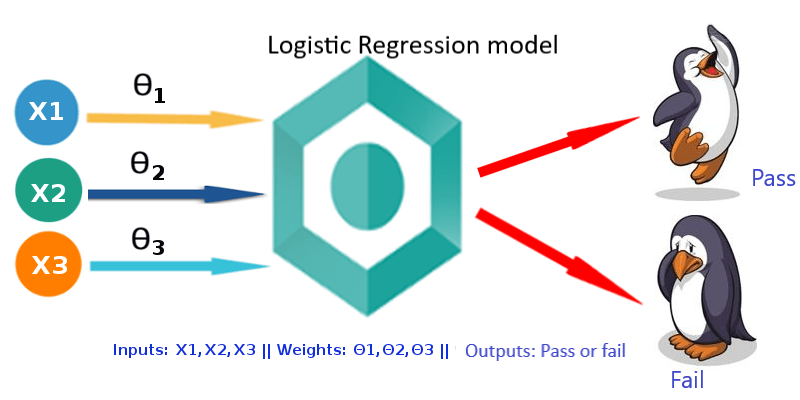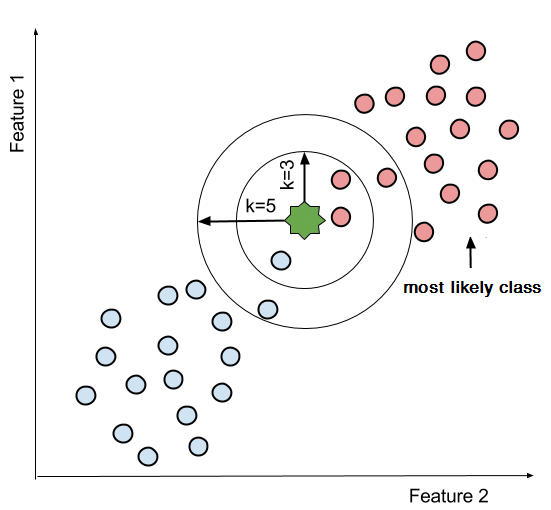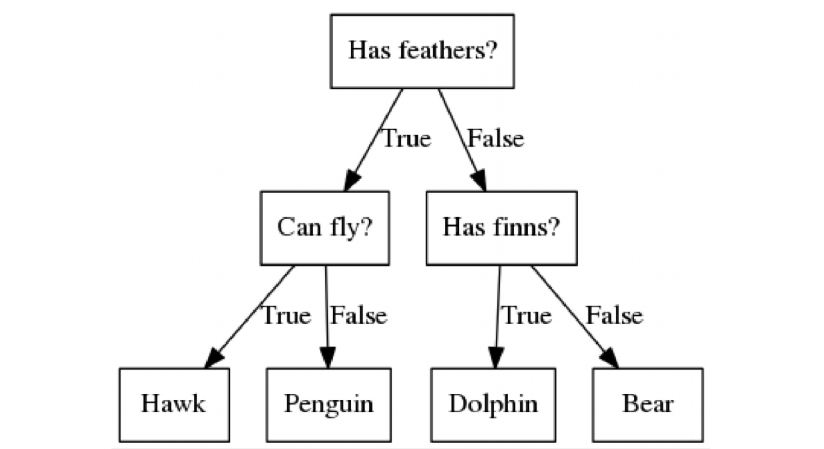# How is Classification used in Data Science?

Get FREE domain for 1st year and build your brand new siteIn this article, we will see how classification is used in Data Science.

• What is classification?
• Uses of classification

# What is classification?

Classification is categorizing data into different classes. Predictive models for classification approximates a function that maps input variables to discrete outputs. The classes are often referred to as labels or target. This is based on making predictions using past examples. We feed some examples where we know what the correct prediction is into the model and the model learns from these examples to make accurate predictions in the future. Hence, this comes under supervised learning.

# Uses of classification

We have a lot of classification algorithms. Broadly they can be divided in to two categories: Linear and non-linear.

• Linear models include logistic regression and support vector machines.
• Non-linear models include K-nearest neighbors, Naive Bayes, Random forest and Decision tree classification.

Logistic regression is a classification algorithm that is used when we need to find relationships between various given attributes to find the likelihood of an outcome. An example of logistic regression is when we need to predict if a student will pass or fail an exam based on the number of hours he/she studied.In real life, logistic regression is used to predict the probability that a patient could develop a particular disease, identify sentiments of reviews, to build predictive models for credit scoring, to suggest equpiments according to a gamer's use for them to buy in a game and also to suggest hotels based on our needs in sites where we book hotels.

When labeled data is difficult to obtain and is expensive, we use K-nearest neighbors algorithm. It classifies new data points based on its similarities to its neighbors. One real life use case of KNN classification is recommender systems. Often we have seen YouTube recommend us some videos based on what kind of content we watch the most. Here, the genre of the content we watch is found using KNN and then the most similar contents or nearest neighbors are displayed as recommendations. Given below is an example of KNN classification.We mostly use Naive Bayes when we are working with text classification and have multiple classes as it converges quicker and consumes less training data. This algorithm works on the Bayes' theorem and is also known as a probabilistic classifier. One real world use case is classifying whether a text article is about technology, sports, politics are any other category. This is known as document classification. Here, the features used to classify documents may be presence or absence of certain keywords.

While the Decision tree algorithm can be used for both regression and classification, it is mostly preferred for solving classification problems. Since it shows a tree like structure, the logic behind it can be easily understood and it mimics the way human think before taking a decision. It can handle all types of data and does not require encoding or normalization. In real world, decision tree classifiers are mostly used as ensembles (bagging and boosting) and are not used alone as they are not the best models. Given below is an example of a decision tree to classify different living organisms.Similar to the case if regression, even in classification, a random forest classifier is used when we do not want our decision tree implementation to overfit on a certain dataset.